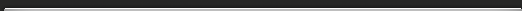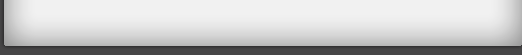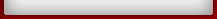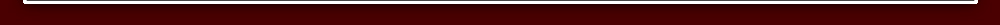Tutorial 1

## Tutorial

Use the formula ALL FROM 9 AND THE LAST FROM 10 to perform instant subtractions.
For example 1000 - 357 = 643
We simply take each figure in 357 from 9 and the last figure from 10.So the answer is 1000 - 357 = 643
And thats all there is to it!
This always works for subtractions from numbers consisting of a 1 followed by noughts: 100; 1000; 10,000 etc.
Similarly 10,000 - 1049 = 8951For 1000 - 83, in which we have more zeros than figures in the numbers being subtracted, we simply suppose 83 is 083. So 1000 - 83 becomes 1000 - 083 = 917

Exercise 1 Tutorial 1
Try some yourself:
1) 1000 - 777 =
2) 1000 - 283 =
3) 1000 - 505 =
4) 10,000 - 2345 =
5) 10,000 - 9876 =
6) 10,000 - 1011 =
7) 100 - 57 =
8) 1000 - 57 =
9) 10,000 - 321 =
10) 10,000 - 38 =